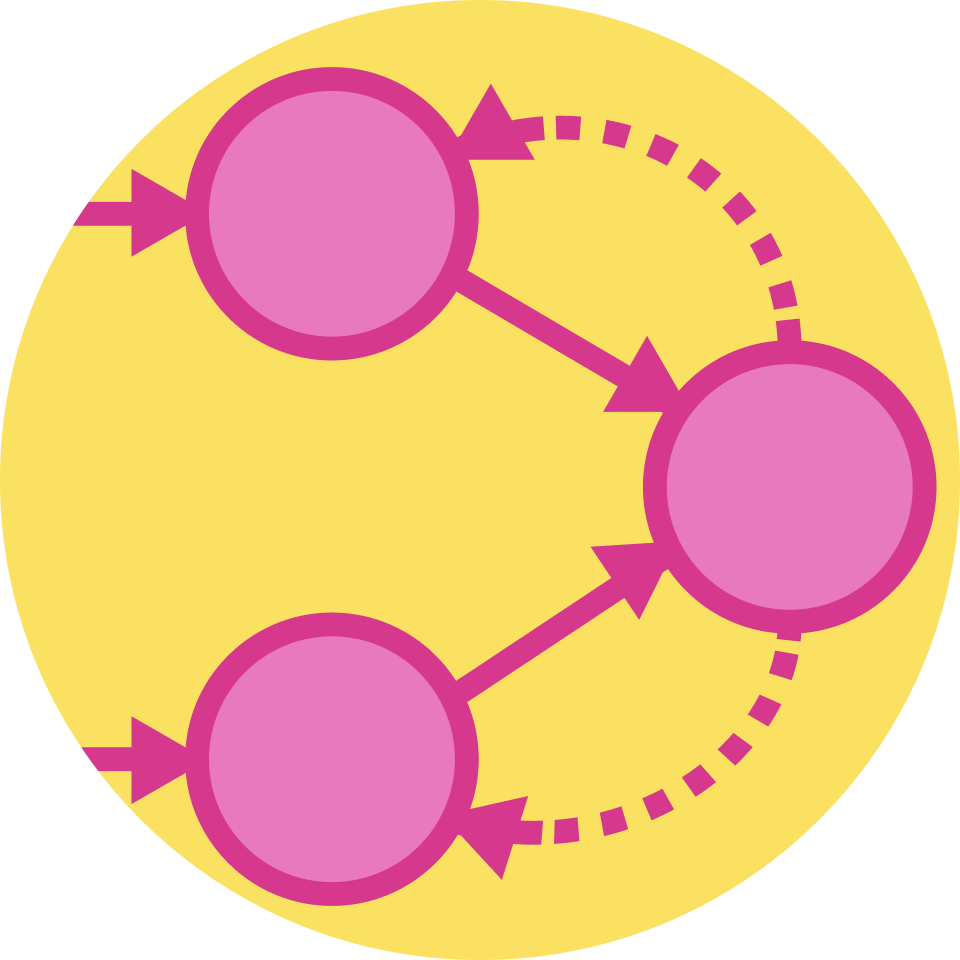## Artificial Neural Networks

A quick dive into a cutting-edge computational method for learning.

Learning Problems for Neural Networks

Computationally Modeling The Brain

Computational Models of The Neuron

Vectors for Neural Networks

Matrices for Neural Networks

Optimization for Neural Networks

Perceptrons as Linear Classifiers

Perceptron Learning Algorithm

Dealing with Perceptron Limitations

Basics and Motivation

Practical Example

Multilayer Perceptron - Model Complexity

Backpropagation - Updating Parameters

Backpropagation

Convolutional Neural Networks - Overview

Convolutions and Striding

Convolutional Neural Networks - Pooling

Applications and Performance

Recurrent Neural Networks

Training Recurrent Neural Networks

Long Short-Term Memory

Stochastic Neural Networks

Variational Autoencoders

Word2Vec

Reinforcement Learning

### Course description

This course was written in collaboration with machine learning researchers and lecturers from MIT, Princeton, and Stanford. This interactive course dives into the fundamentals of artificial neural networks, from the basic frameworks to more modern techniques like adversarial models. You’ll answer questions such as how a computer can distinguish between pictures of dogs and cats, and how it can learn to play great chess. Using inspiration from the human brain and some linear algebra, you’ll gain an intuition for why these models work – not just a collection of formulas. This course is ideal for students and professionals seeking a fundamental understanding of neural networks, or brushing up on basics.

### Topics covered

• Backpropagation
• Convolutional Networks
• Linear Classifiers
• LSTM
• Optimization
• Perceptron
• Recurrent Networks
• Reinforcement Learning
• Stochastic Networks
• Variational Autoencoders

### Prerequisites and next steps

You'll need mastery of algebra. A basic understanding of calculus and probability will be helpful.

### Prerequisites

• Computer Science Fundamentals
• Applied Probability